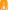638

## Our Rating

6.0Design
5.0Usability
8.0Creativity
6.3Average
##### TAGS
###### Description

Exercise your brain with several series of mental calculations! Just write (draw) the numbers or symbols to solve the equation. In type A, you must calculate the proposed equations (addition, subtraction, division and multiplication). In type B, you must indicate if the left equation is smaller, larger or equal to the right equation. Each part is a series of 20 equations to be solved as soon as possible.